# Solving and Graphing Linear Inequalities Solving OneStep Linear

• Slides: 18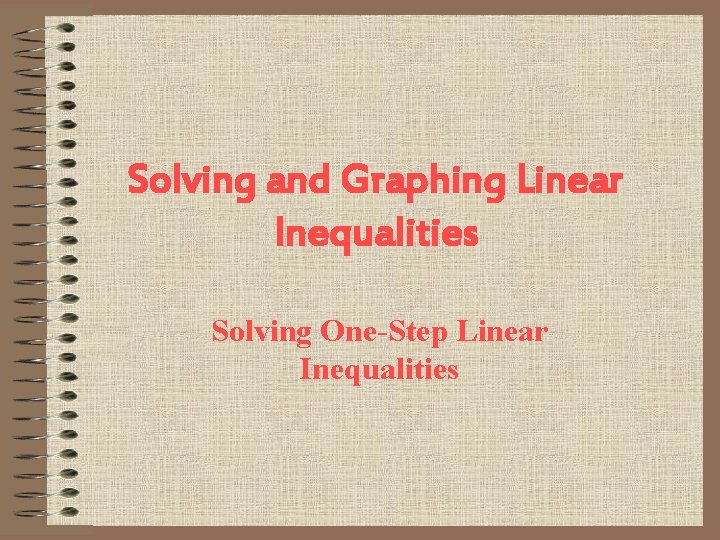Solving and Graphing Linear Inequalities Solving One-Step Linear InequalitiesWhat’s an inequality? • • Is a range of values, rather than ONE set number An algebraic relation showing that a quantity is greater than or less than another quantity. Speed limit:Symbols Less than Greater than Less than OR EQUAL TO Greater than OR EQUAL TO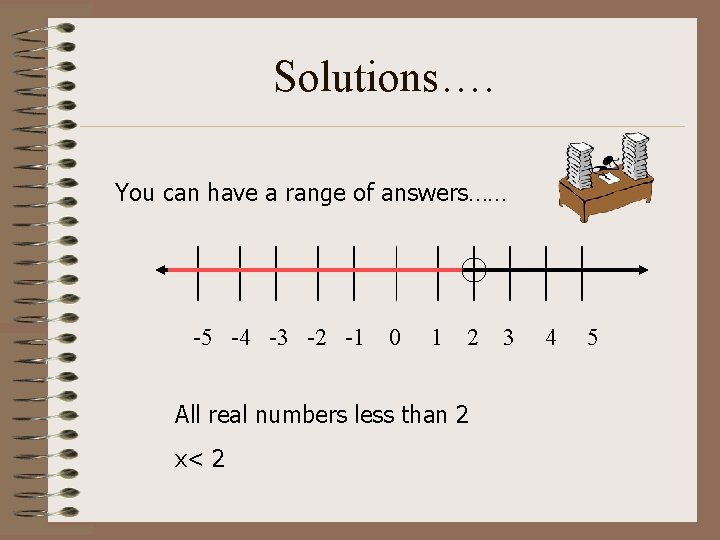Solutions…. You can have a range of answers…… -5 -4 -3 -2 -1 0 1 2 All real numbers less than 2 x< 2 3 4 5Solutions continued… -5 -4 -3 -2 -1 0 1 2 All real numbers greater than -2 x > -2 3 4 5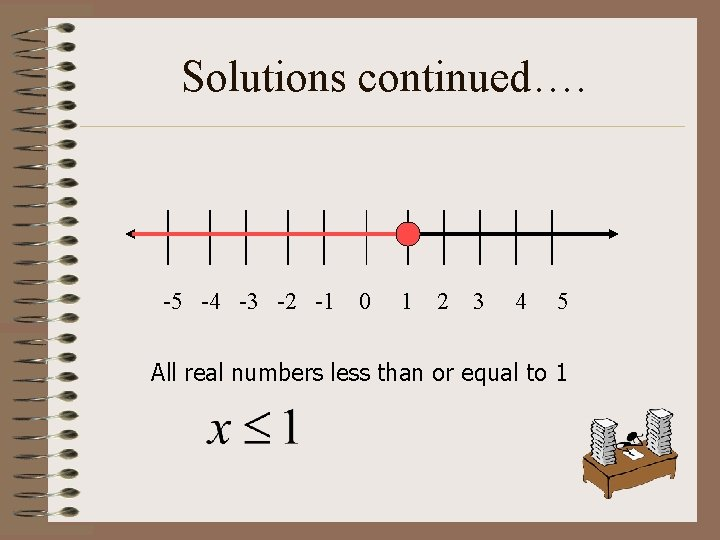Solutions continued…. -5 -4 -3 -2 -1 0 1 2 3 4 5 All real numbers less than or equal to 1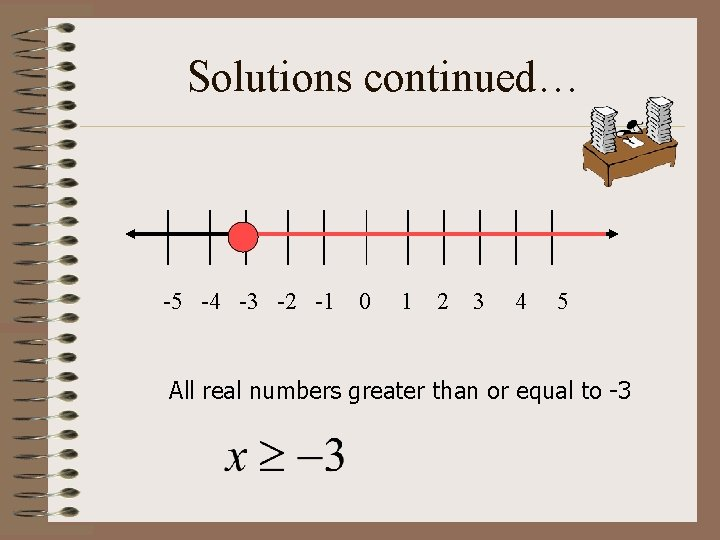Solutions continued… -5 -4 -3 -2 -1 0 1 2 3 4 5 All real numbers greater than or equal to -3Did you notice, Some of the dots were solid and some were open? -5 -4 -3 -2 -1 0 1 2 3 4 5 Why do you think that is? If the symbol is > or < then dot is open because it can not be equal. If the symbol is or then the dot is solid, because it can be that point too.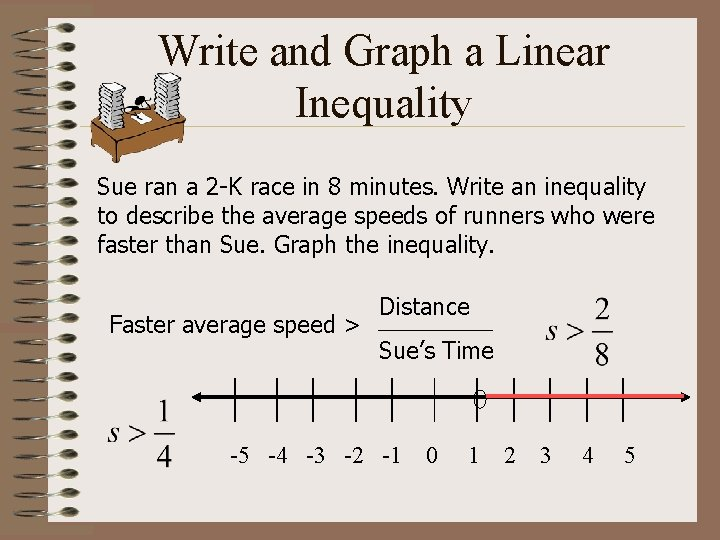Write and Graph a Linear Inequality Sue ran a 2 -K race in 8 minutes. Write an inequality to describe the average speeds of runners who were faster than Sue. Graph the inequality. Faster average speed > Distance Sue’s Time -5 -4 -3 -2 -1 0 1 2 3 4 5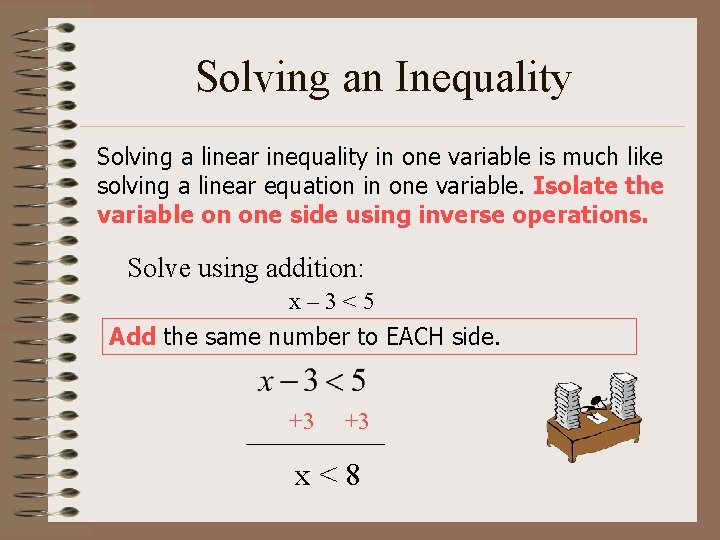Solving an Inequality Solving a linear inequality in one variable is much like solving a linear equation in one variable. Isolate the variable on one side using inverse operations. Solve using addition: x– 3<5 Add the same number to EACH side. +3 +3 x<8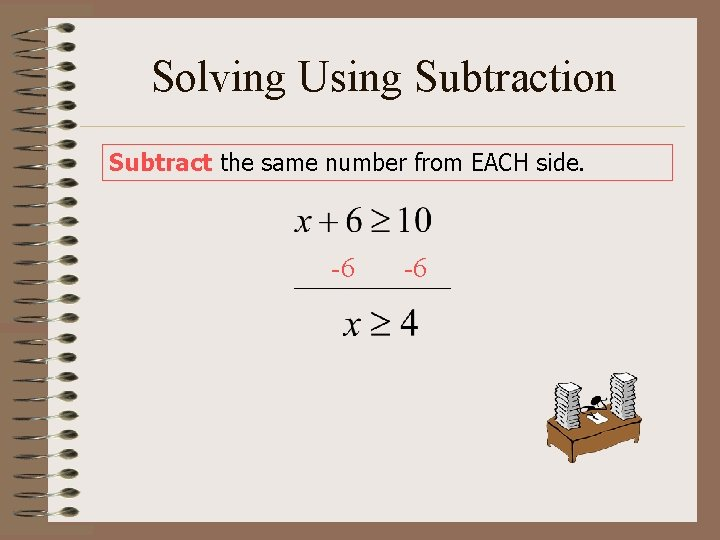Solving Using Subtraction Subtract the same number from EACH side. -6 -6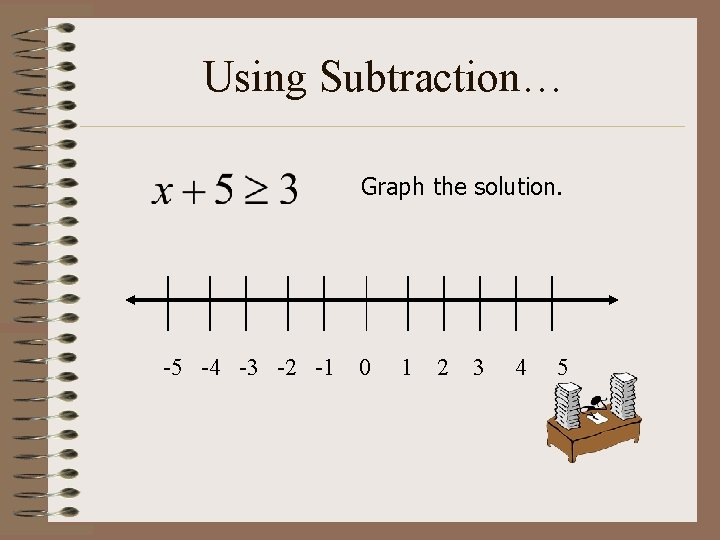Using Subtraction… Graph the solution. -5 -4 -3 -2 -1 0 1 2 3 4 5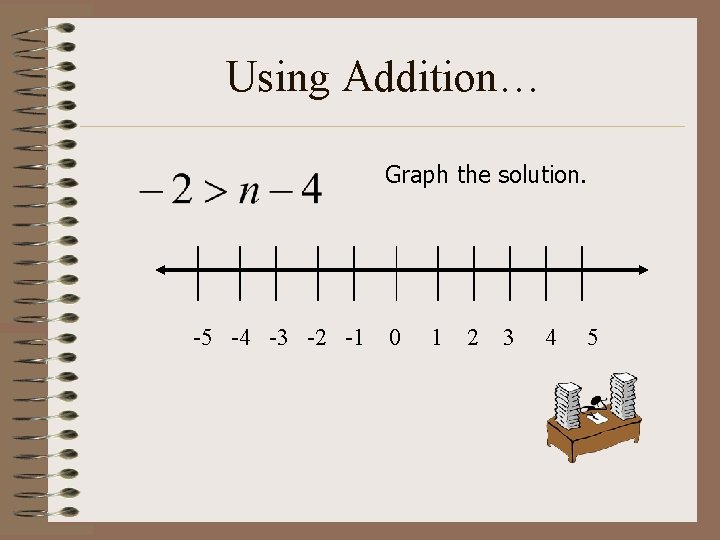Using Addition… Graph the solution. -5 -4 -3 -2 -1 0 1 2 3 4 5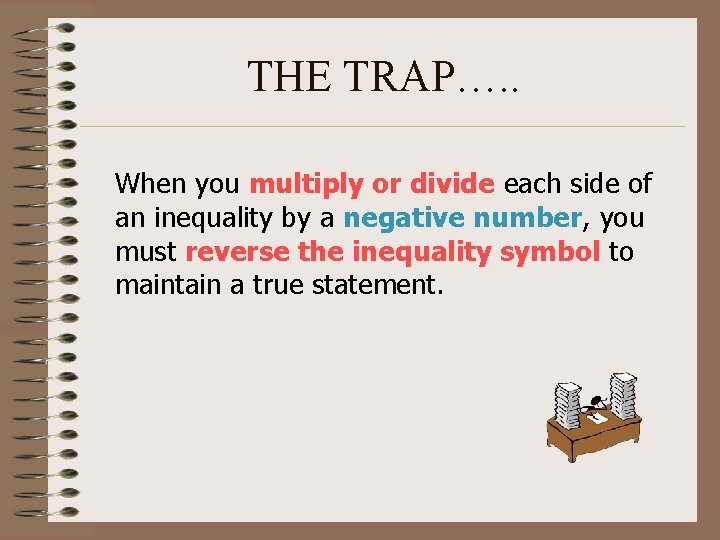THE TRAP…. . When you multiply or divide each side of an inequality by a negative number, you must reverse the inequality symbol to maintain a true statement.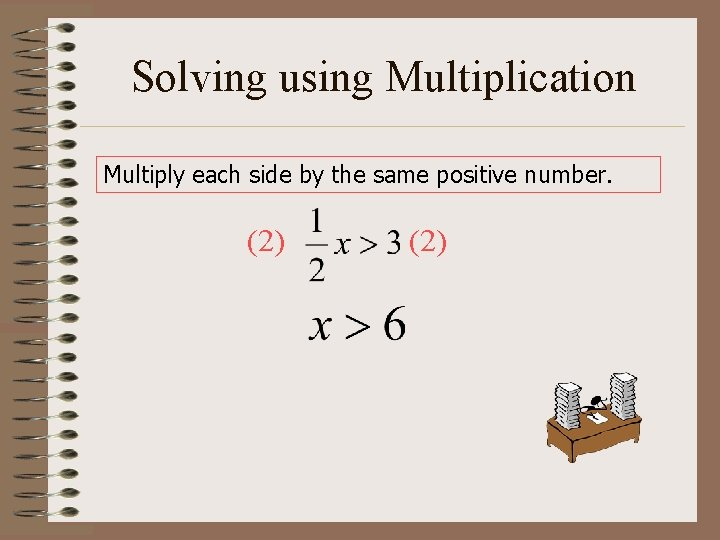Solving using Multiplication Multiply each side by the same positive number. (2)Solving Using Division Divide each side by the same positive number. 3 3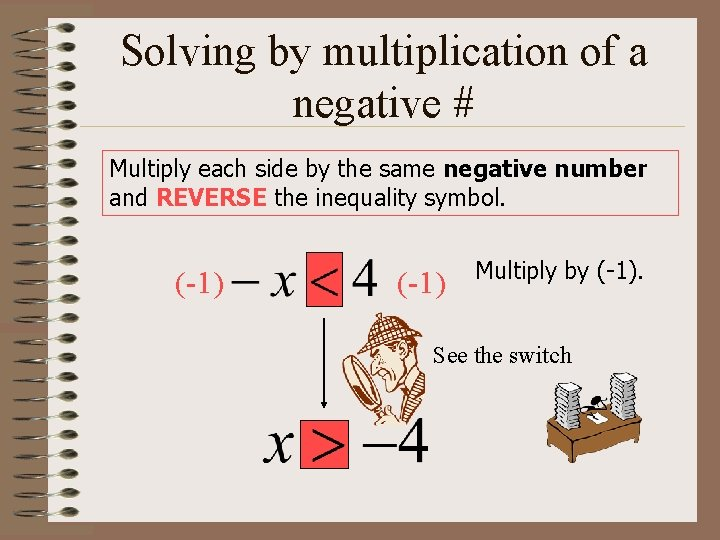Solving by multiplication of a negative # Multiply each side by the same negative number and REVERSE the inequality symbol. (-1) Multiply by (-1). See the switchSolving by dividing by a negative # Divide each side by the same negative number and reverse the inequality symbol. -2 -2